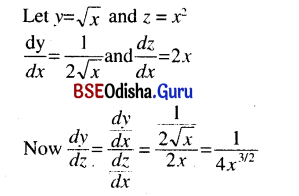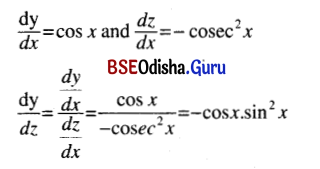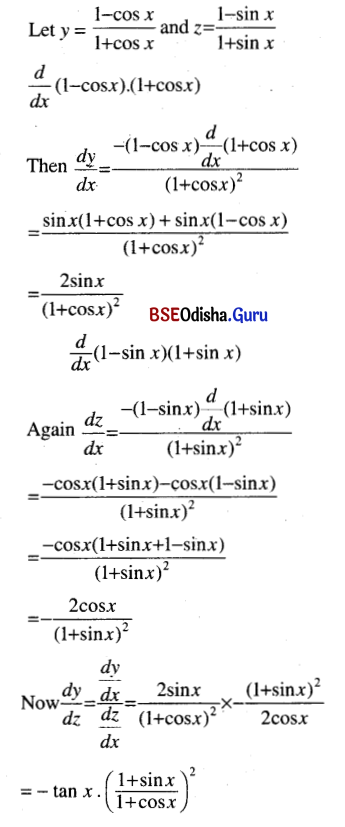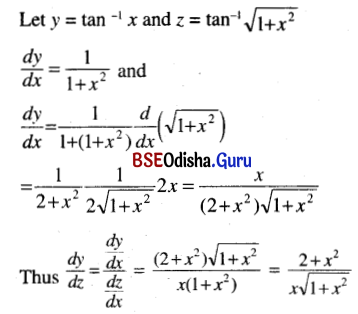# CHSE Odisha Class 12 Math Solutions Chapter 7 Continuity and Differentiability Ex 7(i)

Odisha State Board Elements of Mathematics Class 12 CHSE Odisha Solutions Chapter 7 Continuity and Differentiability Ex 7(i) Textbook Exercise Questions and Answers.

## CHSE Odisha Class 12 Math Solutions Chapter 7 Continuity and Differentiability Exercise 7(i)

Differentiate.
Question 1.
√x w.r.t x2.
Solution:Question 2.
sin x. w.r.t. cot x.
Solution:
Let y = sin x and z = cot xQuestion 3.
$$\frac{1-\cos x}{1+\cos x}$$ w.r.t $$\frac{1-\sin x}{1+\sin x}$$
Solution:Question 4.
tan-1 x w.r.t. tan-1 $$\sqrt{1+x^2}$$
Solution:Question 5.
sin-1 $$\left(\frac{2 x}{1+x^2}\right)$$ w.r.t cos-1 $$\left(\frac{1-x^2}{1+x^2}\right)$$
Solution:
Let y = sin-1 $$\frac{2 x}{1+x^2}$$ and z = cos-1 $$\frac{1-x^2}{1+x^2}$$
Then y = 2 tan-1 x and z = 2 tan-1 x
So y = z
∴ $$\frac{d y}{d z}$$ = 1.